# Find number of months between two dates in Excel using formula

July 22, 2022
1.6K Views
0

A free Office suite fully compatible with Microsoft OfficeIt is a very valuable function to use in excel and it provides employees or workers some relief in their work regarding the monthly process of material in companies. This process also facilitates the corporate-level sectors in their work.

To calculate the number of months between two dates easily, you can use several steps and a formula procedure to follow.

NOTE: We find the number of months between two dates through these steps and the formula below

FORMULA: =DATEDIF(start_date,end_date,m)

1) Find the data below by which we can easily learn how to find the number of months between two dates.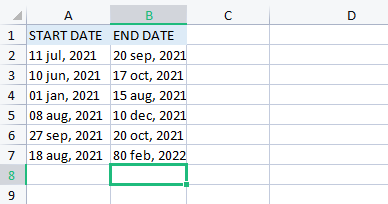2) After the data entry of the start date and end date, we need to add the result section in the C bar where our result of several months between two dates will appear.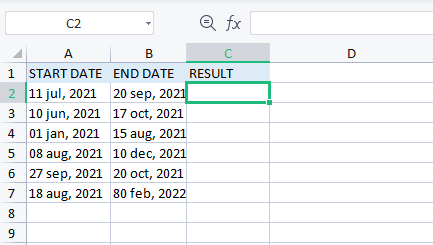3) Our third step is to place the formula in C2 to find the number of months between two dates.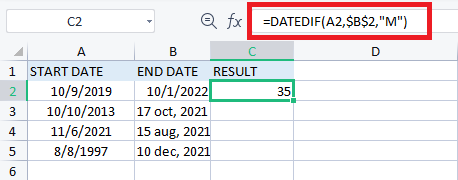This is how we get the result of a number of months between two dates.

4) Our final step regarding this function is to copy the formula up to C6 by which all the numbers of the month will appear in their respective boxes.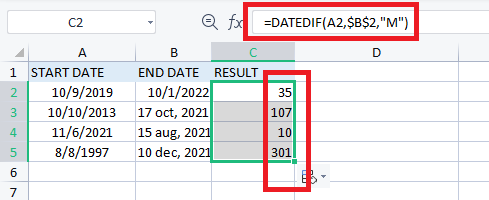NOTE: The above formula will only give you the total number of months between two dates.

How to find a number of months and days between two dates in Excel.

This function is also very helpful to users and it also performs under a few steps and with the help of a formula.

NOTE: This function will only perform when the end date will be greater than the start date.

Steps to find several months and days between two dates in excel are as follows.

1) This function of excel is very similar to finding the number of months between two dates. First, we perform the data which requires.2) The second step is to form a result box where the month and days will appear in C2.3) The third step is to place the formula in the C2 bar where the result of months and days will appear after pressing the enter key.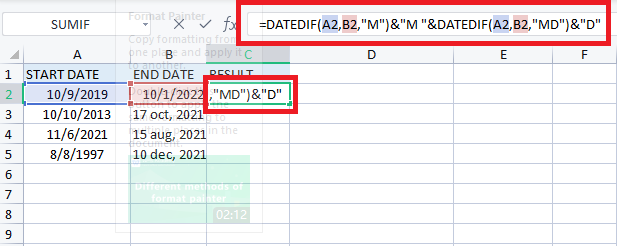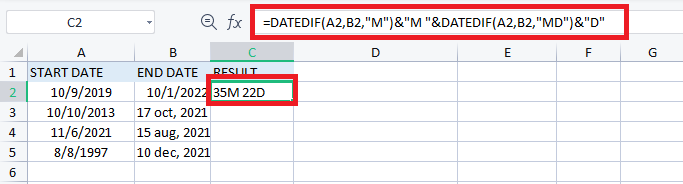4) Last step is to copy the formula up to C5 all the results will appear in their respective boxes.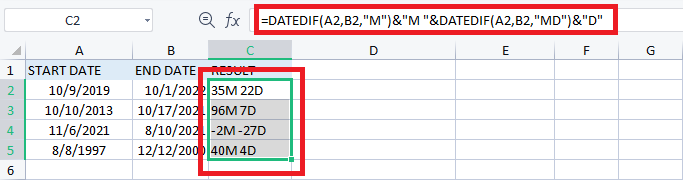Congratulations you successfully find several months between two dates in excel.

How to find several number of months between two dates in Excel using YEARFRAC Function.

Another method to get several months between two dates is by using this YEARFRAC function.

This also requires some steps and a formula which are given below.

1) The First step is that we require data as above examples.

##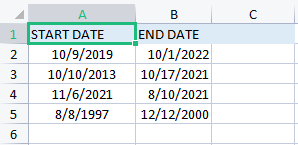2) The Second step is to form a result box where we place the formula and find the months.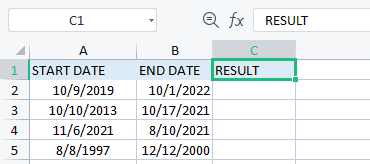3) In the third step we need to place the formula in C2 to find the number of months and the formula which we use here is: =YEARFRAC(A2, B2)*12By implying this formula, we find this result which is highlighted.

4) Our last step here is to copy the formula up to the C5 and all the results will appear and the formula will be copied.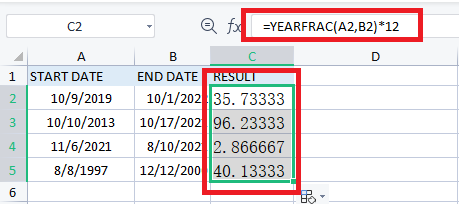Congratulations by following these all steps you find the number of months between two dates easily.

You can also find this feature in the latest excel versions 2016/2018/2019 spreadsheet, where you can easily find the right way to find several months between two dates in excel.